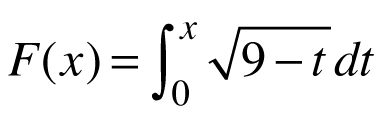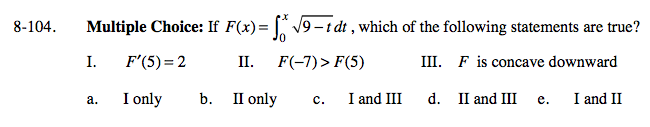### Home > CALC > Chapter 8 > Lesson 8.3.1 > Problem8-104

8-104.
1. Multiple Choice: If, which of the following statements are true? Homework Help ✎

1. F ′(5) = 2

2. F(−7) > F(5)

3. F is concave downward

 a. I only b. II only c. I and III d. II and III e. I and III. Recall that the derivative of an integral gives you the original function (FTC, part 1).

Which is greater?

III. The derivative of the integral, F(x), gives you the original function, f(x). So, the derivative of the original function will give you the 2nd-derivative of F(x).